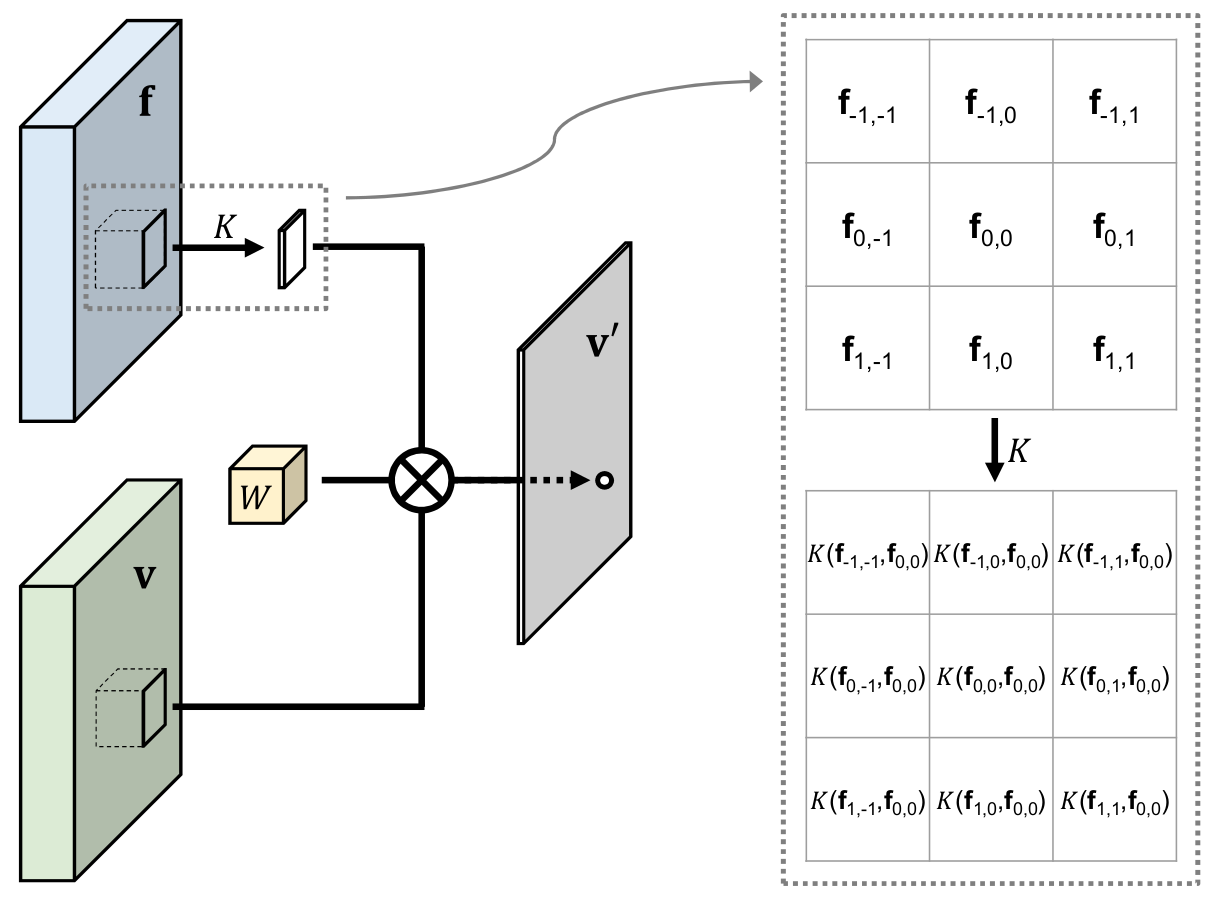## Publication

@inproceedings{su2019pixel,
author    = {Hang Su and
Varun Jampani and
Deqing Sun and
Orazio Gallo and
Erik Learned-Miller and
Jan Kautz},
title     = {Pixel-Adaptive Convolutional Neural Networks},
booktitle = {Proceedings of the IEEE Conference on Computer
Vision and Pattern Recognition (CVPR)},
year      = {2019}
}


## Summary

Convolutions are the fundamental building block of CNNs. The fact that their weights are spatially shared is one of the main reasons for their widespread use, but it also is a major limitation, as it makes convolutions content agnostic.

We propose a pixel-adaptive convolution (PAC) operation, a simple yet effective modification of standard convolutions, in which the filter weights are multiplied with a spatially-varying kernel that depends on learnable, local pixel features. PAC is a generalization of several popular filtering techniques and thus can be used for a wide range of use cases. Specifically, we demonstrate state-of-the-art performance when PAC is used for deep joint image upsampling. PAC also offers an effective alternative to fully-connected CRF (Full-CRF), called PAC-CRF, which performs competitively, while being considerably faster. In addition, we also demonstrate that PAC can be used as a drop-in replacement for convolution layers in pre-trained networks, resulting in consistent performance improvements.

General formula of PAC $$\hat{\mathbf{I}}_p = \frac{1}{Z}\sum_{q \in nbr(p)} \mathbf{I}_q M_q W(q-p) K(\mathbf{f}_p, \mathbf{f}_q) + \mathbf{b}$$ Normalization options
• mask only $$Z_p = \sum_q M_q$$
• $K$ only $$Z_p = \sum_q K(\mathbf{f}_p, \mathbf{f}_q)$$
• mask & $K$ $$Z_p = \sum_q M_q K(\mathbf{f}_p, \mathbf{f}_q)$$
A few special cases
• Convolution $$Z=1, K=1$$
• Pooling $$Z=\frac{1}{\sum K}, W=1, K(:)=1$$
• Bilateral filtering & ConvCRF [Teichmann and Cipolla 2018]
• (no need of normalization when used in ConvCRF)

Choice #1: $$\mathbf{f}=(r,g,b), Z=\frac{1}{\sum K}, W=G^{xy}, K(\mathbf{f}_p, \mathbf{f}_q)=\exp\left(-\frac{1}{2} \|\mathbf{f}_p-\mathbf{f}_q\|^2\right)$$

Choice #2: $$\mathbf{f}=(r,g,b,x,y), Z=\frac{1}{\sum K}, W=1, K(\mathbf{f}_p, \mathbf{f}_q)=\exp\left(-\frac{1}{2} \|\mathbf{f}_p-\mathbf{f}_q\|^2\right)$$

• Detail-preserving Pooling [Saeedan et al. 2018]
• Choice #1 (symmetric kernel): $$Z=\frac{1}{\sum K}, W=1, K(f_p, f_q)= \alpha + \left(\sqrt{(\tilde{f}_p-f_q)^2+\epsilon^2}\right)^\lambda$$

Choice #2 (symmetric kernel): $$Z=\frac{1}{\sum K}, W=1, K(f_p, f_q)= \alpha + \left(\sqrt{\max(0,\tilde{f}_p-f_q)^2+\epsilon^2}\right)^\lambda$$

Notice the use of channel-wise kernel ($K(f_p, f_q)$ instead of $K(\mathbf{f}_p, \mathbf{f}_q)$) and additional filtering ($\tilde{f}_p$ instead of $f_p$).

## Acknowledgements

Hang Su and Erik Learned-Miller acknowledge support from AFRL and DARPA (agreement# FA8750-18-2-0126) and the MassTech Collaborative grant for funding the UMass GPU cluster.

## References

• Marvin T. T. Teichmann, Roberto Cipolla, "Convolutional CRFs for Semantic Segmentation", arXiv:1805.04777, 2018
• Faraz Saeedan, Nicolas Weber, Michael Goesele, Stefan Roth, "Detail-Preserving Pooling in Deep Networks", CVPR 2018